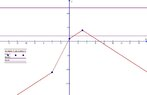# Modulus problem with a negative sign

Nousher Ahmed
In a video, a person discussed how to solve modulus problems with a negative sign. This is the link of that video lecture.

He showed two methods to solve the problem. The first method is commonly used. Later he showed another method where he used a number line and a graph.

Unfortunately, I couldn't understand his explanation properly. What is the original name of the second method he discussed in that video? If I could learn the actual name of the second method, I could googled to learn more about that.

skeeter
He is just plotting the function $f(x) = |x+4| - |x| - |x-3|$ using the coordinate values $(-4,f(-4))$, $(0,f(0))$, and $(3, f(3))$. He selects two more x-values, one > 3 and the other < -4, and evaluates the function at those other two to predict the behavior of the graph.

Note the graph's highest y-value is at $(3, f(3))$ ... it never gets as high as 12, indicating no solution to the equation.

Unfortunately, he doesn't say what to do if, say, $f(x) = 2$ instead of $f(x)=12$. Still, one can see by the graph there would be two possible solutions.Nousher Ahmed
@skeeter , Will you please say what we could do if f(x)=2 instead of f(x)=12?

And probably for f(x)=12, still there might be two possible solutions. Will you please say what those solutions are, and how to find out those solutions?

skeeter
f(x) = 12 has no solutions ... note the graph of f(x) does not intersect the horizontal line y = 12.

If f(x) = 2, I would find the linear equation of the line between the points $(0,f(0))$ and $(3,f(3))$, set it equal to 2 and solve for x.

Do the same for the linear equation of the line between the points $(3,f(3))$ and say, $(5,f(5))$ and follow the same procedure.

Nousher Ahmed
f(x) = 12 has no solutions ... note the graph of f(x) does not intersect the horizontal line y = 12.

If f(x) = 2, I would find the linear equation of the line between the points $(0,f(0))$ and $(3,f(3))$, set it equal to 2 and solve for x.

Do the same for the linear equation of the line between the points $(3,f(3))$ and say, $(5,f(5))$ and follow the same procedure.
Thanks a lot for your cordial help.